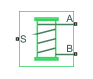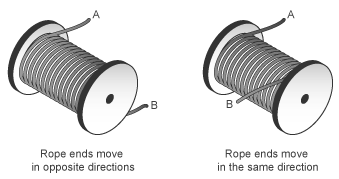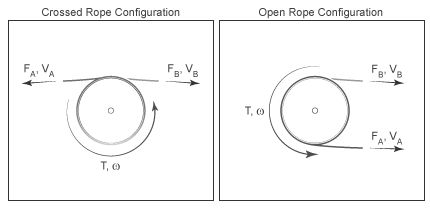# Rope Drum

Power transmission system with tightly wound rope around cylindrical drum

•Libraries:
Simscape / Driveline / Couplings & Drives

## Description

The Rope Drum block represents a power transmission system where a rope is tightly wound around a cylindrical drum at a sufficient tension level to prevent slipping. You can configure the rope so that the ends point in the same or opposite directions. You can set the direction that the ends move with the Rope windup type parameter:

• If the rope ends point in the same direction, they translate in opposite directions.

• If the rope ends point in opposite directions, they translate in the same direction.

The equations refer to rope ends A and B, as illustrated by the figure.During normal operation, a driving torque causes the cylindrical drum to rotate about its axis of symmetry. The rotating drum transmits tensile force to the rope ends, which translate with respect to the drum centerline. The relative direction of translation between the two rope ends depends on the setting of the Rope windup type parameter. However, a positive drum rotation always corresponds to a positive translation at port A.

The block assumes that each rope end remains taut during simulation because slack rope ends do not transmit force. You can set the block to warn you if the rope loses tension.

You can optionally set parameters for:

• Drum inertia

• Viscous friction at the drum bearing

The net torque acting on the cylinder satisfies the force balance equation

`$T={F}_{B}·R·\delta -{F}_{A}·R+\mu ·\omega ,$`

where:

• T is the net torque acting on the drum.

• FA and FB are the external forces pulling on rope ends A and B.

• R is the drum radius.

• μ is the viscous friction coefficient at the drum bearings.

• ω is the drum angular velocity.

• δ is the rope windup type according to the parameter settings:

• When you set Rope windup type to `Ends move in the same direction`, δ = -1.

• When you set Rope windup type to ```Ends move in the opposite directions```, δ = +1.

The figure shows the equation variables.The translational velocities of the two rope ends are functions of the drum radius and angular velocity. Each translational velocity is equal to the tangential velocity of a point at the drum periphery according to the expressions

`$\begin{array}{c}{V}_{A}=-\omega \text{\hspace{0.17em}}·R\\ {V}_{B}=+\omega \text{\hspace{0.17em}}·R\end{array}$`

where:

• VA is the translational velocity of rope end A.

• VB is the translational velocity of rope end B.

### Assumptions and Limitations

• The block ignores slip at the rope-drum contact surface.

• The block ignores rope compliance.

## Ports

### Conserving

expand all

Mechanical rotational conserving port associated with the drum shaft.

Mechanical translational conserving port associated with rope end A.

Mechanical translational conserving port associated with rope end B.

## Parameters

expand all

### Rope Winding

Distance between the drum center and its periphery. The drum periphery coincides with the contact surface between the drum and the rope.

Relative direction of the translation motion of the two rope ends, A and B, where B is the following end.

Option to generate a warning when a rope end becomes slack. A rope end becomes slack when the net tensile forces responsible for keeping it taut fall below zero. Because slack cables do not transmit force, the simulation results may not be accurate when the rope loses tension.

### Drum Dynamics

Linear damping coefficient in effect at the drum bearing. This coefficient determines the power losses due to viscous friction at a given drum angular velocity.

Option to account for drum inertia.

• `No inertia` — Select this setting if drum inertia has a negligible impact on driveline dynamics. This setting sets drum inertia to zero.

• ```Specify inertia and initial velocity``` — Select this setting if drum inertia has a significant impact on driveline dynamics.

Moment of inertia of the drum about its rotation axis. In the simple case of a solid cylindrical drum, the moment of inertia is

`$I=\frac{1}{2}M{R}^{2},$`

where M is the drum mass and R is the drum radius.

#### Dependencies

To enable this parameter, set Inertia to ```Specify inertia and initial velocity```.

Angular velocity of the drum at the start of the simulation.

#### Dependencies

To enable this parameter, set Inertia to ```Specify inertia and initial velocity```.

## Version History

Introduced in R2012a